# [Example 2] Suspended Material Transport in a Simple Bed Flume

In this section, we perform the following computations using a simple curved flume with straight inlet out let parts. The Cross section of the flume is composed with a compound channel in which both the low water channel and the flood plane with moveable bed. Then flood plane is located only left side of the low water channel. The experiment was carried out by CTI Engineering Co. Ltd. on behalf of Civil Engineering Research Institute of Cold Region . A movie taken from a drone during the experiment is shown in Figure 53, and the experimental condition and plane and cross sectional view pictures are shown in Figure 54.

The computational exercises in this section is conducted as the following procedure.

• Flow and bed deformation by Nays2DH until the bed reaches an equilibrium state

• Quasi 3-dimensional flow field by Nays2d+

• Tracer tracking by GELATO. Check the effect of turbulent diffusivity by changing parameter

## Calculation of Flow and bed deformation by Nasy2DH

### Select a Solver

From the iRIC startup screen, click [Create New Project], and select [Nays2dH iRIC3x 1.0 64bit] in the Figure 55.

A window titled as「Untitled- iRIC 3.x.xxxx [Nays2DH iRIC4X 1.0 64bit]」appears.

### Grid Creation

Select from the main menu [Grid]->[Select Algorithm]. Then a window appears as Figure 57, select [2d arc grid generator (Compound Channel)] and click [OK].

In the [Groups] of the [Grid Creation] window, set parameters of, [Channel shape], [Cross section], [Additional Channel] and [Roughness and fixed/moveable bed] as, Figure 58 , Figure 59 , Figure 60 , and Figure 61 , respectively.

When you finished all the settings of the grid creating condition, click [Create Grid] in the above grid creating condition windows, e.g. Figure 61. After clicking [Create Grid] button, you will be asked [Do you want to map?], then answer [Yes], and the computational grid is created. ( Figure 62 )

Put check marks in [Grid], [Cell Attributes] and [Fixed or Moveable bed] in the object browser, Figure 63 appears with the fixed bed part in red and the moveable bed part shown in blue.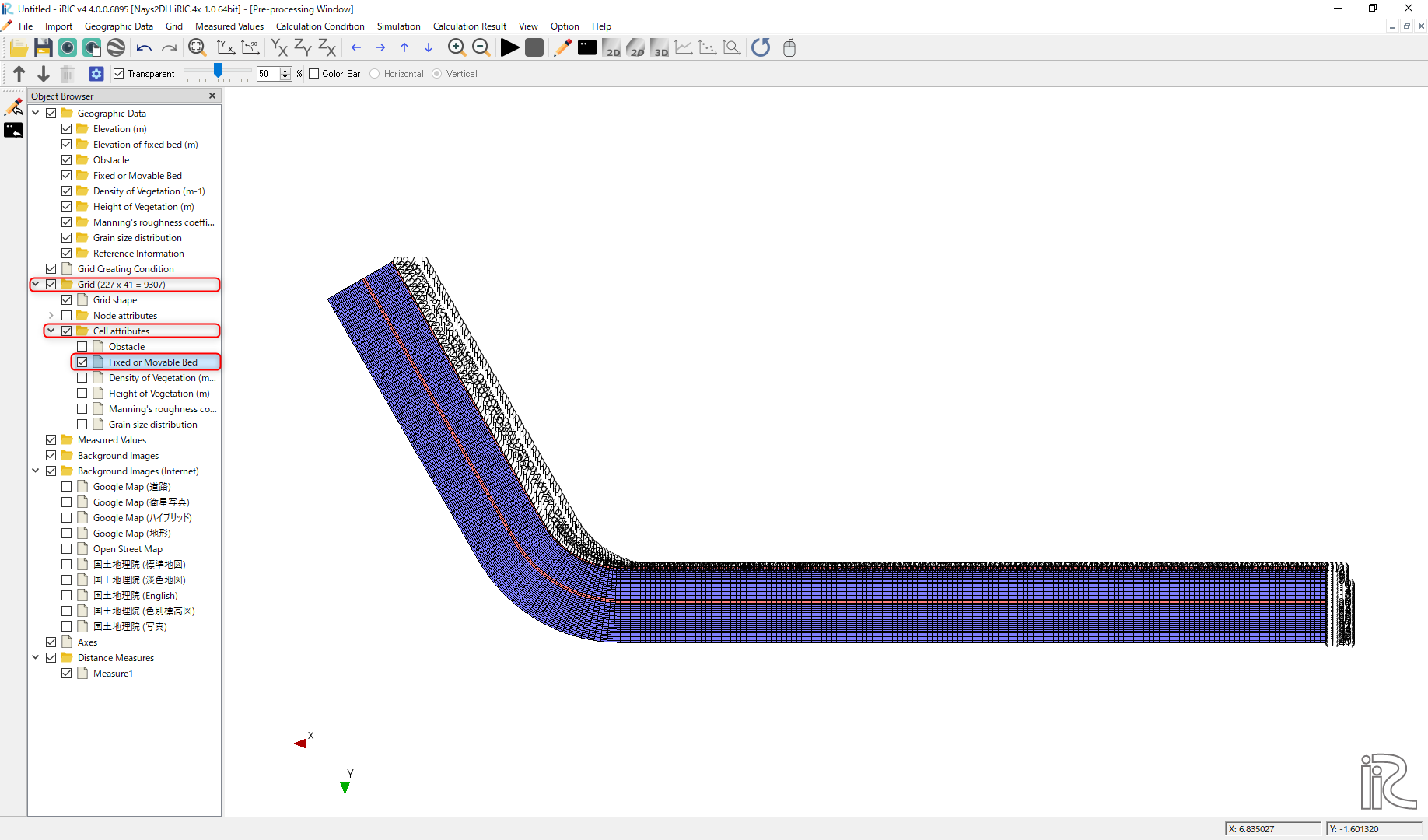Figure 63 : Grid Shape with Fixed and Moveable bed Colored

The red part of the fixed bed along the boundary between the low water channel and the flood plane is assumed to be a revetment, in this grid creating tool, however, since the revetment in the actual experiment is only the bend part plus short length of upstream and downstream. So, as shown in Figure 64, focus [Fixed or Moveable bed], and right-click on a straight section of the revetment part (in this case, the red section upstream of grid number 101) and change the attribute to [Moveable bed], and press [OK].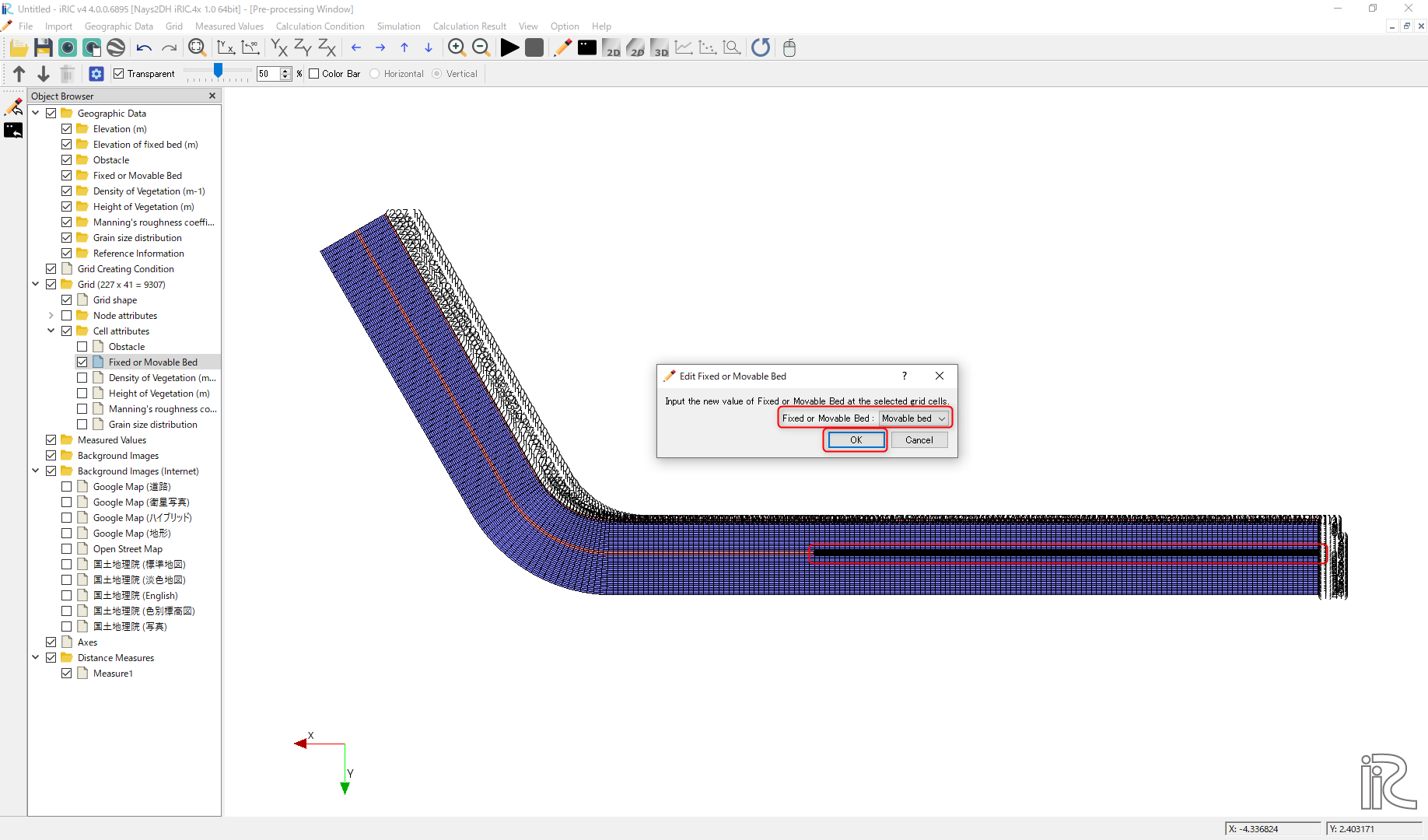Figure 64 : Change attribute from fixed bed to moveable bed

Since the downstream end is the fixed bed, set the attribute of the downstream end cells into [Fixed Bed], by expanding and rotating, as demonstrated in Figure 65.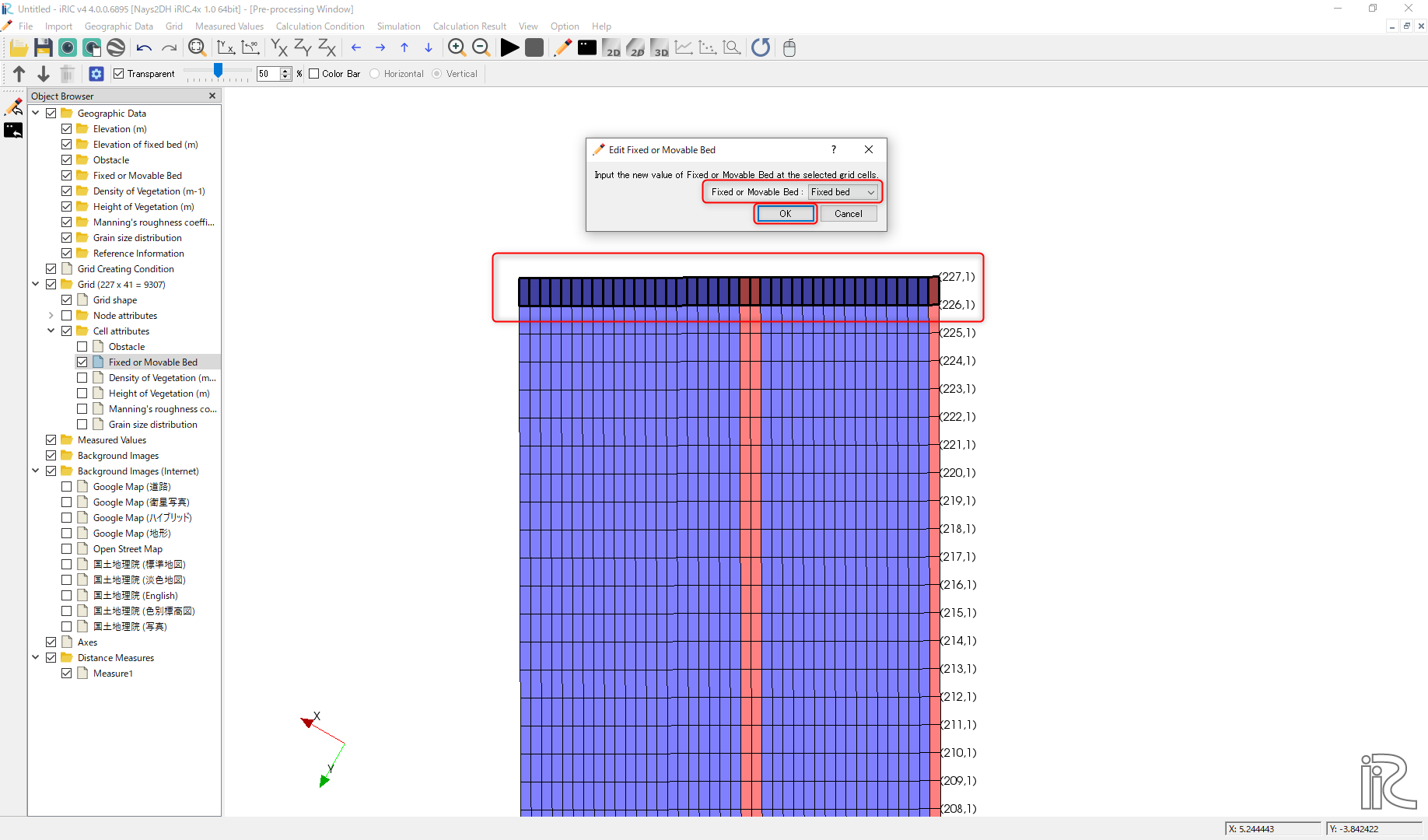Figure 65 : Change downstream end cell attribute to fixed bed

### Setting Computational Condition

Show the [Calculation Condition] window by selecting [Calculation Condition]->[Setting], and in the [Group] of [Solver Type], [Boundary Condition], [Time] and [Bed Material] , set the parameters, as Figure 66 , Figure 67 , Figure 68 , and Figure 69, respectively.

In addition, in the [Boundary Condition] setting of Figure 67, press  of [Time series of discharge at upstream end ……], and set [Time] and [Discharge] hydrograph data in the [Time series of discharge at upstream end ……] window as Figure 70, and press [OK].

When you finished the settings of all the computational condition parameters, press [OK] in the [Calculation Condition] window.

### Run Nays2DH

Before executing the Nays2DH, select [File]->[Save as Project] and save the project. Here we save the project as a name of [Nays2DH_flow_bed] (Figure 71)

From the main menu, select [Simulation]->[Run], then a window asking [Do you want to save?] appears as Figure 72. Then press [Yes], save as a project, and the computation starts running as Figure 73.

When the computation finished, save the results by selecting [Calculation Result]->[Save], from the main menu.

### Display the Calculation Results

Open a [Post Processing Window] by selecting [Calculation Result]->[Open new 2D Post-Processing Window] as Figure 74.

In the object browser of the [Post Processing Window], put check marks in [iRICZone], [Scalar(node)] and [ElevationChange(m)], right click [ElevationChange(m)] to show [Property] and press it, open [Scalar Settings], and set parameters as Figure 75.

In the object browser, put check marks in [Arrow] and [Velocity(m)], right click [Arrow], show [Property] and press it, open [Arrow Setting Window] as Figure 76, and set parameters as marked with red squares in the Figure 76.

Put the [Time Scale Bar] back to zero, select [Animation]->[Srart/Stop] to start animation as Figure 77.

As shown in Figure 78, it is shown that the bed elevation change reached an equilibrium.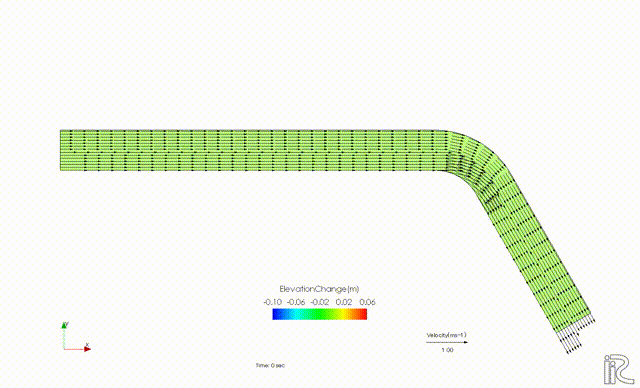Figure 78 : Animation of velocity vectors and bed elevation changes

### Export the Computational Results

In order to use the calculated bed elevation as an boundary conditions for the quasi-3D flow calculation by Nays2d+ in the next section, we export the calculated results to a text file. As shown in Figure 79, select [File]->[Export]->[Calculation Result].

When the [Export Calculation Result] setting window (Figure 80) is appeared, choose [Format] as [Topography Files(*.tpo)].

The output folder can be any name, and uncheck the checkbox at [All timesteps], and set [Start] and [End] as 10,800. Then click [OK] to complete the export of the calculation Results Figure 81.

The exported calculation results are stored in the specified folder. As shown in Figure 82, many files contain different values as water depth, velocity, sediment transport rate, riverbed elevations, and so on, however, since only the riverbed elevation is used for the flow calculations in the next section, all files except [Result_1_Elevation(m).tpo] can be deleted.

## Quasi-3D Flow Calculation by Nays2d+

### Selecting a Solver

From the iRIC startup screen, click [Create New Project], and select [Nays2d+] in the Figure 83, and press [OK].

### Importing Computational Grid, Channel Bed Elevation and Mapping

#### Importing Grid

From the main menu, select [Import]->[Grid], and choose [Case1.cgn] in the folder of [Nays2DH_floe_bed] which was created in the previous section. While importing, a warning as Figure 84 is coming out, press [Yes], and complete importing grid (Figure 85).

#### Import Bed Elevation

From the main menu, select [Import]->[Geographic Data]->[Elevation](Figure 86).

In the import file selection window, Figure 87, assign the file [Results_1_Elevation(m).tpo], which was exported from Nays2dH calculated results in the previous section.

Figure 88 appears, but if there is no particular need to thin out the data, you can leave it as it is, and press [OK] to complete the import the [Bed Elevation] (Figure 89).

#### Execute Mapping

The imported bed elevation data is mapped onto the imported computational grid. Select [Grid]->[Attribute Mapping]->[Execute] as Figure 90.

As Figure 91, you will be asked which [Geographic Data] to be mapped. Put check mark in the box of [Elevation(m)], and press [OK].

When the mapping is completed, press [OK] as Figure 92.

### Setting Calculation Condition for Nays2d＋

In the window of [Calculation Condition] which appears when you select [Calculation Condition]->[Setting], set parameters in the [Groups] of [Discharge and downstream water surface elevation], [Time and bed erosion parameters], [Boundary Condition], [Other computational parameters] and [3D Velocity Profile] as, Figure 93, Figure 94, Figure 95, Figure 96, and Figure 97, respectively.

In addition, while in the settings of the [Discharge and downstream water surface elevation], Figure 93, press  and set discharge data in in the [Time series of discharge and downstream stare] setting window as Figure 98.

When you finish setting all the calculation condition, press [OK] in the [Calculation Condition] window.

### Execute Nays2d+

We will skip the explanation of how to executing Nays2d+ because it is exactly same as other solvers. However, it is recommended that you save the project before running the calculation. In this case, we save the file to a project named [Nays2d+Flow].

The results are saved in a CGNS file named [Case1.cgn], which will be used for the tracer tracking computation of GELATO as input data. Be sure to save the result using [Calculation Result]->[Save] even when the calculation is finished. (Figure 100).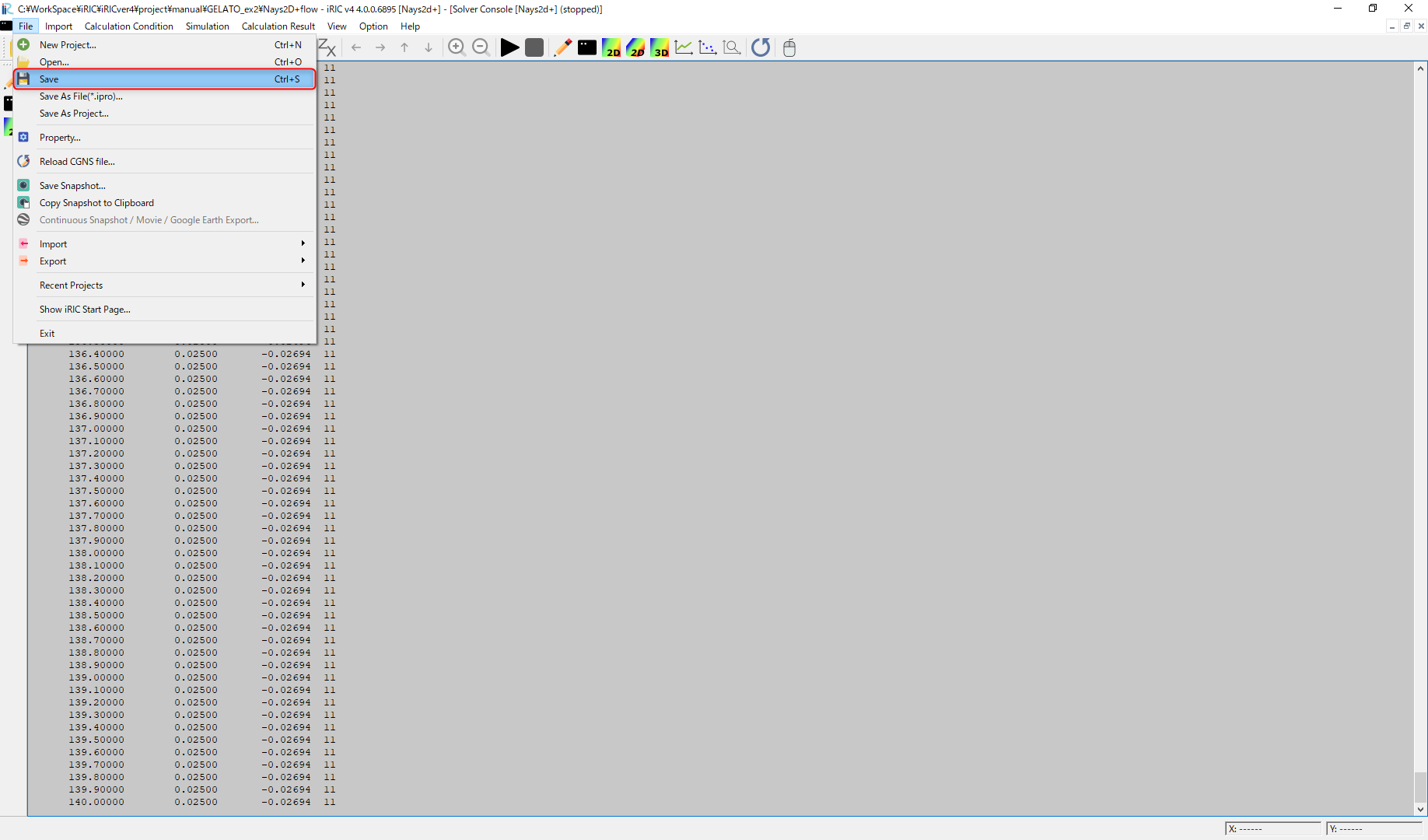Figure 100 : Save the Results of the Computation (Don’t Forget!)

## Tracer Tracking by GELATO

### Select a Solver

From the iRIC startup screen, select [New Project], and in the solver selection screen appears. Select “GELATO” and click “OK” (Figure 101).

### Import Grid

Right click [Grid(No Data)] and select [Import] as Figure 102.

From the [Select Import File] window as Figure 103, choose [Case1.cgn] in the folder [Nays2d+Flow] which is produced by the [Nays2d+] calculation in the previous section.

Press [Yes] button when warning message is coming out as Figure 104, and the grid import is completed as Figure 105.

### Tracer Tracking Simulation by GELATO

#### Setting Simulation Condition

From the main menu bar, when you select [Calculation condition]->[Setting]. [Calculation Condition] window appears, and in this window, set parameters in the [Groups] of [Basic Settings], [Normal Tracers Supplying Condition] and [Diffusion Condition], as Figure 106, Figure 107, and Figure 108, respectively. In this section, we first perform tracer tracking without considering the effect of sub-grid turbulence.

In addition.Figure 100 The [CGNS file to read the flow calculation results] in the [CGNS file to read the flow calculation results] is the same as the one in the previous section [Flow calculation with Nays2d+]. Select [Case1.cgn] in the [Nays2d+Flow] project folder where you saved the results of ( Figure 103)

In addition, the [Flow information input file] in Figure 106, is the same file with the [Case1.cgn] which was produced by the flow simulation of [Nays2d+] in the previous section (Figure 110).

#### Run GELATO

From the main menu, select [Simulation]]->[Run], then you will be asked to save project as usual, save project as recommended. ( Figure 111).

In the Figure 112, either [Save as file (*.ipro)] or [Save as Project] will do, but in this example, the file is saved as [GELATO1].

When the computation starts, Figure 113 appears, and when the computation finishes, Figure 114 appears. Press [OK] to finish computation.

#### Showing the Results of GELATO

From the main menu, select [Calculation Result]->[Open new 2D Post Processing Window], and the calculation results are shown (Figure 115)

Since the orientation of the Figure 115 is the opposite to the experimental image shown at the beginning of this chapter Figure 53, press the 90° rotation mark twice to rotate 180° (Figure 116).

Since the [Time] display is so small that it’s hard to see, select [Time]->[Properties] in the object browser (Figure 117), display [Time Setting] and set the font size appropriately large (Figure 118).

As shown in Figure 119, put time bar back to 0, and from the main menu, select [Animation]->[Start/Stop], then the animation starts( Figure 120).

There is almost no diffusion and the tracers are just flowing straightly.

#### Comparison of the Turbulent Diffusivity

Select [Calculation Condition]->[Setting] and open [Calculation Condition] window. As shown in Figure 121, set in the [Group]->[Diffusion Condition], [Diffusivity Correction]->[Yes] and set the value [A=10] of the [Diffusivity Parameter]

In the same manner, if we do the simulation with [A=5], [A=10] and [A=50], the results becomes as Figure 123, Figure 124 and Figure 125.

When we compared with the experimental results of the Figure 53, it seem that the case with A=10, Figure 124, is the closest to the experiment.

#### Cloning of the Tracers

In the main menu, select [Calculation Condition]->[Setting] to show [Calculation Condition]. In the [Calculation Condition] window, select [Tracer Cloning and Amalgamation], set parameters as Figure 126. Select [Diffusion Condition] and set [A=10] and press[OK] as Figure 127. Then execute the GELATO solver by choosing [Simulation]->[Run], and show the results (Figure 128).

The spread range of the tracers in Figure 53 is close to the diffusion range of the green dye in the experimental movie. The number of tracers appears to be enormous, but if you put check marks in [Particles]->[Scalars]->[Generations] in the object browser, generations of the tracers are displayed as Figure 129.

When this is animated, it becomes as Figure 130.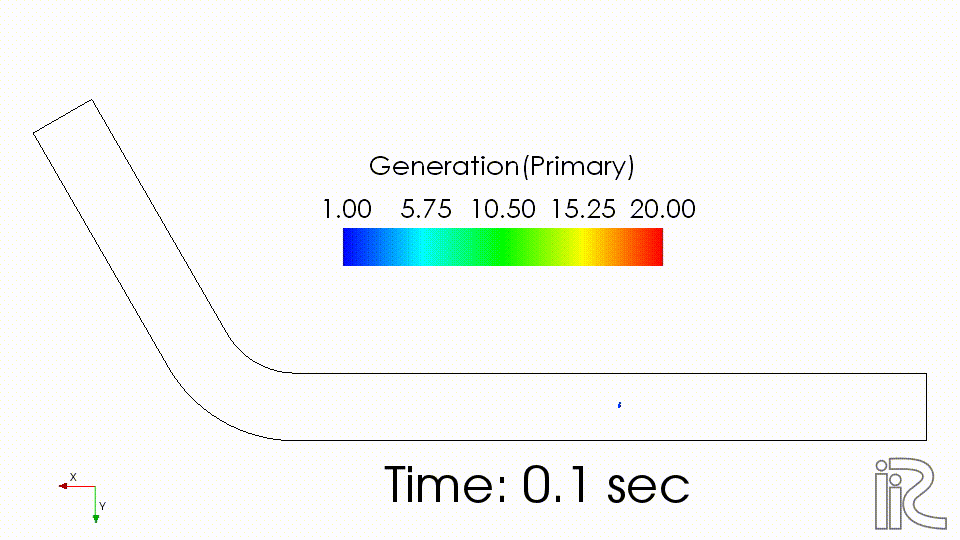Figure 130 : Tracers Clone Animation(Maximum 20 Generations, A=10, Color-coded View)

As described in Overview , the substantial weight in the 10th generation is W=0.00195, and in the 20th generation is W=0.00000195. Therefore, Figure 129, the concentrations of the tracers of green, yellow, red, etc. are logarithmically lower than that of the central blue tracers. To see the real concentration, the substantial concentration in each cell is visualized by the following procedure.

1. Uncheck the check box at [Scalar] in the object browser (Figure 131).

2. Put check mark at [Scalar(Cell Center)] and [Weighted numbers of tracers] in the Object Browser (Figure 132).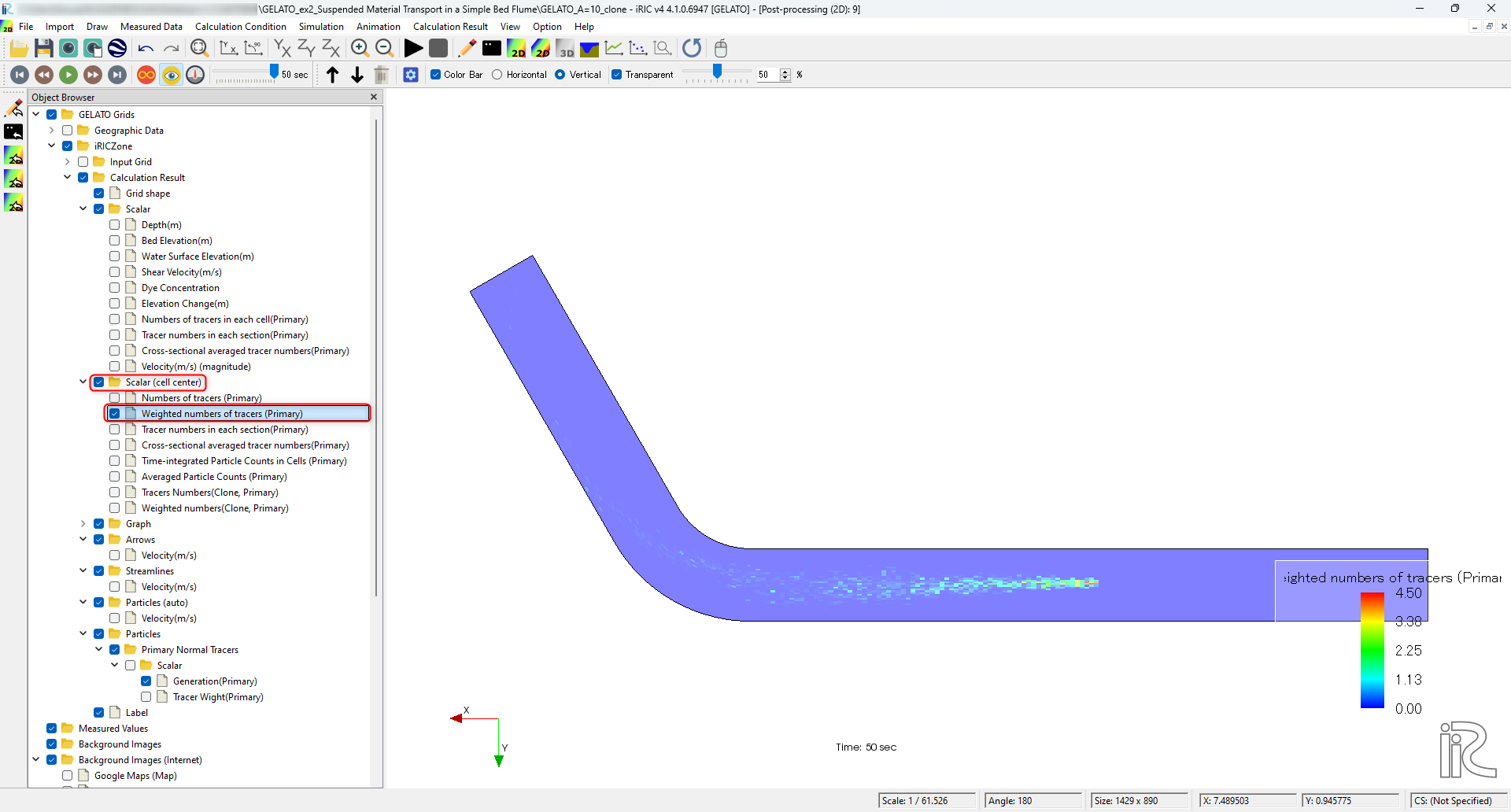Figure 132 : Put check mark at [Weighted numbers of tracers]
1. Right click [Weighted numbers of tracers] and press [Property]

1. In the [Scalar Setting] window, setting as shown Figure 131.

5. As shown in Figure 135, put time bar back to 0, and from the main menu, select [Animation]->[Start/Stop], then the animation starts( Figure 136).

The diffusion situation is similar to that of the green dye in the experimental movie of Figure 53.

### Flow Visualization using Tracer Cloning

Flow visualization using tracer cloning is shown in this section. In the main menu, click [Calculation Condition], and set parameters in the [Group] of [Normal Tracers Supplying Condition] and [Tracer Cloning and Amalgamation] as Figure 137 and Figure 138, respectively, and press [OK].

Figure 137

Then after running the GELATO solver. in the [Object Browser], remove check mark from [Weighted numbers of tracers], put check marks in boxes at [Particles], [Scalar] and remove the check mark form the [Generation].

From the main menu, select [Animation]->[Srat/Stop], and the animation with evenly distributed tracers in the whole channel is visualized.

### Swimming Fish Simulation

Set the following parameters in the [Computation of Fish Motion] in the [Calculation Condition] window menu followed by selecting [Calculation Condition]->[Setting] in the main menu.

After setting these parameters, run the solver by [Simulation]->[Run]. Once close the existing [2D Post-processing 2D window], open a new [2D Post-processing 2D window], put check mark on [Polygon]->[Fish]->[Type] as Figure 145, and select [Animation]->[Start/Stop]. Then Figure 146 is played.

## Driftwood Tracking by NaysDW2 and Visualization

In this section, driftwood tracking simulation by NaysDW2 (Nays Driftwood 3D) is shown.

### Select a Solver

From the iRIC startup screen, click [Create New Project], and select [NaysDw2(Simple 2D Driftwood Tracker)] as shown in Figure 147, and press [OK].

### Import Computational Grid

As shown in Figure 148, from the [Object Browser], right click [Grid(No data)], and press [Import]

When the file selection window appears, select [Case1.cgn] in the [Nays2d+Flow] folder in which the computational results of the [Nays2d+] stored. (Figure 149)

Neglect the waring message as Figure 104, press [Yes], and the grid importing is completed (Figure 151).

### Setting Condition

From the main menu, select [Calculation Condition]->[Setting],and set the calculation condition as follows.

In the [Calculation Condition] window, press file selection bar as Figure 152.

In the [Select File] window, Figure 153, select [Case1.cgn] which contains the calculation results of the [Nays2d+] in the previous section.

Set other parameters in [Basic Setting] as Figure 154.

Set parameters in [Driftwood Feeding Condition] as Figure 155.

Set [DEM Coefficients] parameters as Figure 156, and press [OK].

### Run Driftwood Simulation

From the main menu, select [Simulation]->[Run] as Figure 157.

When you are asked [Do you want to save?] as Figure 158, press [Yes] and save the project.

As Figure 159, when you are asked [How to save the project], in this example, select [Save as project], and press [OK]. Choose an empty folder to save project, and press [Select Folder].

When the calculation starts, Figure 160 is displayed, and Figure 161 is appear when the calculation ends. Then click [OK] to finish calculation.

### Visualization of driftwood motion

From the main menu, select [Calculation Result]->[Open New 2D Post-processing Window] as Figure 162.